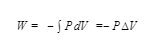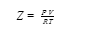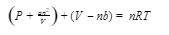Thermodynamics Help – Get answers to thermodynamics questions!

## Thermodynamics basics – topics & concepts:

### Ideal gas-

It is a hypothetical gas composed of many particles which has negligible intermolecular force of attractions.

This ideal gas is useful because it obeys the ideal gas equation.

That is,     PV=nRT     where R is gas constant

Assumptions taken -

The volume of the molecule is negligible as compared to the volume of the container.

All collisions are elastic.

No force of attractions exist between the molecules.

### Molecular statistics–

It is the branch of thermodynamics that explains the molecular properties to predict the macroscopic quantities of compounds.

Here, in the molecular statistics thermodynamics, there is the energy levels of an individual molecule to find the thermodynamic properties of a system that contains non-interacting molecules of a substance.

### Transport phenomena-

It involves the movement or transfer of mass, energy or matter within the thermodynamical system that are not in equilibrium.

• There is a continuous flow of molecules or particles.
• The movement of molecules by random collisions is called diffusion.

There are 3 areas of study

• Heat transfer
• Mass transfer
• Fluids mechanics

### First law of Thermodynamics-

This is also known by “THE LAW OF CONSERVATION OF ENERGY “ which relates that the energy of the universe remains the same.

This stated that the total energy of the universe remain same and describing the relation between internal energy, heat absorbed or released and work done on or by the system.

∆U=Q+W

W = +( positive) WORK DONE ON THE SYSTEM BY THE SURROUNDINGS

W= - (negative) WORK DONE BY THE SYSTEM ON THE SURROUNDINGS

Q = + (positive) Heat absorbed

Q=-(negative) heat released

Reversible work done### Second law of thermodynamics-

First law of thermodynamics defines the quantity of heat transfer but do not give any idea of the direction.

So, by 2nd law – it focuses on the direction to which the heat or energy flows and introducing a term called Entropy for every spontaneous or non spontaneous process.
And states that,

In an isolated system, the entropy of the universe will always increases.

Entropy is Denoted by ∆S

Also, for spontaneous process, entropy is positive and for non spontaneous it is negative.

∆S(universe)  = ∆S(system) +  ∆S (surrounding)

General relation,

∆G= ∆H-T∆S

∆G = Gibbs free energy, ∆H = enthalpy   , ∆S = entropy and T = Temperature

### Third law of thermodynamics-

It states that the entropy of a perfect crystalline solid at absolute   zero is 0

S=k lnW = kln 1 = 0

It means at absolute 0K , All modes of motion (vibration , rotation and translation )stops

### Imperfect gas–

It is also called Real gas which has some known magnitude of intermolecular force of attraction and they occupy a given amount of space and have the ability to interact with each other.

Also, For the determination of deviation of a real gas from the behaviour of an ideal gas , it is expressed by term Z as compressibility factor.The ideal gas equation can be modified into the van der Waal equation to apply to real gas where a and b are constants### Liquids–

It is the state of the matter lies between solids and gases and The attraction between the particles in a liquid keeps the volume of liquid constant.

Some properties of liquids-

• It has fixed volume but no shapes that is why takes the shape of vessel , to which it is contained
• It is incompressible
• It has little space between them, in comparison to the gases.

## Sample Thermodynamics Questions:

Question1: Calculate the Gibbs free energy at 200K when enthalpy and entropy changes are -113 KJ/mol and -145J/Kmol ?

Ans: -83KJ/mol

∆G= ∆H-T∆S
= -113000 – 200 (-145)
= -8300 J/mol

Question2: Calculate the workdone by a piston of a container, which has 200J of internal energy to absorb the 5 joule.

Using first law of thermodynamics,
U=Q+W
U-Q =W=200-5
-195 J Which means, 195j of work is done by the system

Quesion3: Calculate the work done of a reversible system which apply a pressure of 2atm to change the volume from 5L to 10L ?

Reversible work done= -2atm(10-5)

= -10atm Litre                          1atm L=101.325J

-10  101.325 = - 1013.2J

## MCQ:

Q1. Real gases follow ideal gas equation –

A) low temperature and high pressure
B) high temperature and low pressure
C) low temperature only
D) no change in conditions

Ans: B

Q2. In which of the process, the  system is  spontaneous.

A) Freezing of water
B) dissolution Of Iodine in water
C) Burning of a wood
D) Melting of an ice

Ans: D

Q3. At Absolute 0K , entropy of is zero for

A)  Perfectly crystalline solid
B) non crystalline
C) amorphous

## Why choose TutorEye for Thermodynamics Help?

Thermodynamics is a branch of physics that deals with the processes of change in temperature, transformation of energy, relationships between heat and work. The study of thermodynamics gained popularity in the 19th century when engineers wanted to improve the efficiency of the steam engine. The study of thermodynamics focuses mainly on the four laws of thermodynamics.

### Zeroth Law-

According to this law of thermodynamics, if two bodies are in thermal equilibrium with a 3rd system, then they must be in thermal equilibrium with each other.

### First Law-

This stated that the total energy of the universe remains the same and describes the relation between internal energy, heat absorbed or released and work done on or by the system.

### Second Law-

In an isolated system, the entropy of the universe will always increase.

### Third Law–

It states that the entropy of a perfect crystalline solid at absolute  zero is  always zero.

Basic thermodynamics is taught in most engineering degree programs as well as it is a part of the high school physics curriculum. An introductory background to concepts of thermodynamics in high school as well as knowledge of calculus helps one to become successful and make a career in the field of thermodynamics.

Thermodynamics is also a prerequisite for advanced physics courses, like heat transfer, internal combustion engines, gas dynamics etc. Students completing a bachelor’s or master’s course in thermodynamics can lead to an exciting career as a thermodynamics engineer, engineering technician, or an expert thermodynamics physicist.

The application of thermodynamics can be found in the field of engineering from chemical thermodynamics, thermodynamics physics, to aerospace, mechanical and electrical engineering.

Learning Thermodynamics may become a challenge to many high school & college students if the thermodynamics basics are not clear and a strong foundation is not established in the early introductory courses.

Our tutors at TutorEye make thermodynamics basics easy to understand and follow. Breaking down complex thermodynamic questions into a smaller step helps retain the theory and build conceptual clarity.

Use our Physics Formula Sheet for High School Students to check out the thermodynamic formulas and understand the parameters and assumptions used for arriving at the answer.

To gain confidence with laws of thermodynamics and thermodynamics questions, our tutors recommend taking help in solving previous year question papers. Avail written help anytime from anywhere and get the most reliable thermodynamics help.

## How to get Thermodynamics help?

To avail help with assignments, follow these few simple steps:

Step 1: Submit your assignment requirement on a quick-help form for speedy assistance

Step 2: Hire a homework solver depending on the quoted price and promised deadline

Step 3: You can duly vet the handed over assignment before releasing payment from escrow

We firmly believe in providing the best quality assignment help service and handing over plagiarism-free work. You can rely on us to hand over 100% authentic well-written drafts to quickly help you go through the most difficult problems.

At TutorEye, we provide the highest quality and therefore follow a Money Refund policy to ensure quality writing standards at all costs. Unburden your study load by hiring our top homework solvers today!

## Top Thermodynamics Questions:

### How can I learn thermodynamics easily?

Thermodynamics can be fun only if you devote time to fully understand its process and terminologies. To ensure you can learn it easily you must:

• Summarize or jot down points such as that of closed and open systems
• Make use of flowcharts and mind maps as chapters become complex
• Solve problems immediately after you understand a concept
• Understand all experimental facts
• Learn definitions and laws

Yet, if you need mentoring or top guidance in the subject, you can connect with our homework helpers who can easily provide Thermodynamics help. Let’s assist you to chalk out a plan to learn the subject.

### What are the basic concepts of thermodynamics?

We know there are various forms of energy like heat, work etc. Thermodynamic mainly deals with heat and relates the conversion of heat to other forms of energy. It also explains the transfer of heat from one system to another and relates other thermodynamic terms like enthalpy, entropy, free energy etc.

### How many laws are there in Thermodynamics?

According to libretext.org, there are four laws of Thermodynamics. They are as follows:

• The Zeroth Law of Thermodynamics
• The First Law of Thermodynamics
• The Second Law of Thermodynamics
• The Third Law of Thermodynamics

If you need help in understanding these laws or the principles in question, you can avail help from our best academicians. With our help, you can uncomplicate the chapters and Thermodynamics basics in the shortest possible time and get better grades.

### Who gave the laws of thermodynamics?

According to wikipedia, there are many scholars who are associated with different laws of Thermodynamics:

• The Zeroth Law is associated with Fowler who had coined the term while discussing it with B.N. Srivastava and Meghnad Saha
• The First Law was coined by Rudolf Clausius in 1850 and some scholars also credit William Rankine. However there were many others who act as precursors or developed theories regarding this law.
• The coining of Second Law is credited to Nicolas Léonard Sadi Carnot. However, Rudolf Clausius came up with the most prominent classical statements in 1854.
• The Third Law was developed by a chemist named Walther Nernst during 1906-1912

These laws were a result of developed work on previous postulates and theorems given by earlier philosophers and mathematicians. To know more, get help from our certified professionals to uncover the secrets behind these laws and so much more.

### What is thermodynamics ?

Thermodynamics is the branch of physics that deals with the study between heat and energy.

### What are the thermodynamics properties ?

Thermodynamic properties are defined as characteristic of a system capable to  specifying the system's state in the system.

### What are the 1st and 2nd law of thermodynamics?

The 1st law of thermodynamics  that energy neither t be created nor destroyed.  it can only be transferred  from one form to another form.

The 2nd  law of thermodynamics is about the quality of energy. It states that energy is transferred or transformed more and more of it is wasted.

### What are the thermodynamics parameters?

The central concept of thermodynamics is that of energy and the ability to do work.

### Why is it called thermodynamics?

Science of the relationship between heat, work, temperature, and energy is called thermodynamics . thermo(heat) and Dynamics (transfer).

### What is the limitation of thermodynamics?

The first law of thermodynamics  is that it does not say anything about  the direction of flow of heat.

### What is the limitation of the  2nd law  of thermodynamics?

The 2nd law  of thermodynamics only applicable to the closed system.